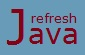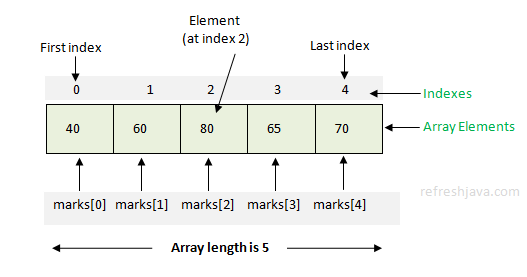# Java Arrays

As we know java provides primitive data types to store single values like `20, 100, 20.5` etc in a variable. What if I need to store multiple values of same data type like `20, 30, 40` or `10.5, 20.4, 30.6` etc in a single variable, one approach could be, create multiple variable and assign single values in each variable. Another easy way is to use arrays provided by java.

An array in java is a container which allows us to store multiple values of same data type in a variable. The syntax of declaring an array is :

``` `DataType`[] arrayName;
`DataType` arrayName[]; ```

You can use any of the above two forms to declare an array in java. For examples :

``` `int`[] intArray; ` // declares an array of integer with name as intArray`
`int` intArray2[]; ` // declares an array of integer with name as intArray2`
`float`[] floatArray; ` // declares an array of float with name as floatArray`
`char`[] charArray; ` // declares an array of char with name as charArray` ```

The `[]` used with data type or array name suggest's that it's an array. The data type of an array can be primitive or non primitive, while the name of array is given as per the programmer's choice. The name must follow the rules and convention given in Identifier-naming-convention section. Arrays of primitive data types stores values while arrays of non primitive data types stores the object references.

### Which approach I should prefer to declare an array ?

Though you can use any of the above form but it's good practice to use first one as it is more meaningful. The code `int[] intArray` itself suggest that variable `intArray` is an `int` type array. Java convention also discourage to use the second form which is `int intArray[]`.

The code given below shows how to declare an array of non primitive data type. Assuming that you have already created a class `MyFirstProgram`. To declare an array of `MyFirstProgram`, use below syntax :

` `MyFirstProgram`[] mfpArray; ` // declares an array of MyFirstProgram class with name as mfpArray` `

## How to initialize array in Java

Java arrays initializes array values in a continuous memory location where each memory location is given an index. You can assign or access the value to that memory location using it's index. The index starts from `0` and goes as `0,1,2,3,4...`

The first approach to create or initialize an array in memory is by using `new` keyword. The code below initializes an array in memory with size as 5 and then assigns values in it using it's indexes.

``` `int`[] marks = new `int`;

marks = 40; ` // initialize first element`
marks = 60; ` // initialize second element`
marks = 80; ` // initialize third element`
marks = 65; ` // initialize fourth element`
marks = 70; ` // initialize fifth or last element`

`// initializing in one line`
`int`[] marks = new `int`[]{40,60,80,65,70};
```## How to access values of array

Each item in an array is called an `element`, and each element of array is accessed by its index. The index of first element is `0`.

``` marks ` // To access element 40`
marks ` // To access element 60`
marks ` // To access element 80`
marks ` // To access element 65`
marks ` // To access element 70`    ```
```  `Min or start index of an array = 0;`
`Max or last index of an array = length of array - 1;`	 ```

### What is length or size of an array ?

The maximum or total number of elements that can be assigned into the array is known as length or size of an array. You can get the length of an array using the `length` property of array.

The second and shortcut approach to initialize an array in memory is by directly assigning array values into array variable like below :

``` `int`[] intArray2 = {20,40,60}; ` // initializes an int array with size as 3`
`int`[] intArray3 = {
20,30,40,
50,60,70,
80,90,100
};` // initializes an int array with size as 9`

` // Do not use comma(,) after last element.`
```

The length of array in above declaration is determined by the number of values given inside the `{}` and separated by comma(,). You can assign the elements of array in one line or multiple line as given above.

### Which approach I should prefer to initialize an array ?

If you already know the elements(values) that need to be assigned in array, you should prefer the 2nd approach as it's more easy. But it's completely your choice to use the one you prefer.

### Can I increase length or size of an array after initialization ?

No, Once an array is created or initialized, the length of array is fixed. You can not increase or decrease length of array after initialization. For eg. if you create any array of length 5 as ```int[] marks = new int;``` You can not access or assign value at 5th index(`marks`) which is 6th element, if you access `marks`, java will throw a runtime exception.

You can also assign one array into other array like below. Just remember in this case if you make any changes in element of one array, that will be reflected in other as well.

``` `int`[] intArray2 = {20,40,60};
`int`[] intArray3 = intArray2; ` // intArray3 refers intArray2`
```

### What if i don't assign a value to a particular index ?

If programmer doesn't specify a value to a particular index of an array, java will itself assign a value to that index as per the data type of array. For eg. `0` for `int`, `0.0` for `double`, `false` for `boolean` etc. For non primitive data type it assigns `null` for that index.

### Can I pass array from one method to other ?

Yes, You can pass array from one method to other method as you pass normal variables.

### Array program in Java

```` class` ArrayInJava
{
`public static void` main(String [] args)
{
`int`[] marks = new `int`;
marks = 40;
marks = 60;
marks = 80;
marks = 65;
marks = 70;

System.out.println(`"Element at index 0 = "`+marks);
System.out.println(`"Element at index 1 = "`+marks);
System.out.println(`"Element at index 2 = "`+marks);
System.out.println(`"Element at index 3 = "`+marks);
System.out.println(`"Element at index 4 = "`+marks);

`// Accessing array elements using for loop`;
System.out.println(`"Accessing array element using for loop ........"`);
`for`(`int` i=0; i<5; i++)
{
System.out.println(`"Element at index "`+i+`" = "`+marks[i]);
}
}
}```

Output:

Element at index 0 = 40
Element at index 1 = 60
Element at index 2 = 80
Element at index 3 = 65
Element at index 4 = 70
Accessing array element using for loop .........
Element at index 0 = 40
Element at index 1 = 60
Element at index 2 = 80
Element at index 3 = 65
Element at index 4 = 70

The program below calculates the average of given integer numbers of an array. It also shows how to use the `length` property of array which returns the length of an array.

```` class` ArrayDemo
{
`public static void` main(String [] args)
{
`int`[] numbers = {40,60,80,65,70};
`double` avgNumber;
`int` total = 0;
`// finding sum of all numbers of array`;
`for`(`int` i=0; i < numbers.length; i++)
{
total = total+numbers[i];
}
avgNumber = total/5;
System.out.println(`"Average of numbers of array = "`+avgNumber);
}
}```

Output:

Average of numbers of array = 63.0

Arrays with single [] brackets is also known as one dimensional array. Arrays discussed in this tutorial is single dimension arrays, for multidimensional arrays refer next section.

★★★
• Array elements starts from index 0, not 1
• If you access array variable name, java will return reference(address) of that variable.
• Accessing any elements outside array index will throw ArrayIndexOutOfBoundsException at runtime.
• A method can return an array as well to calling method.